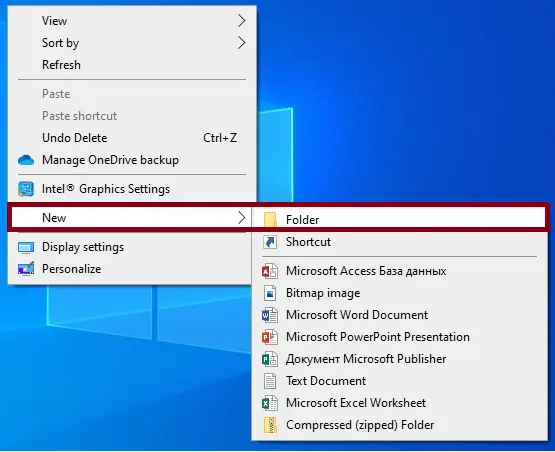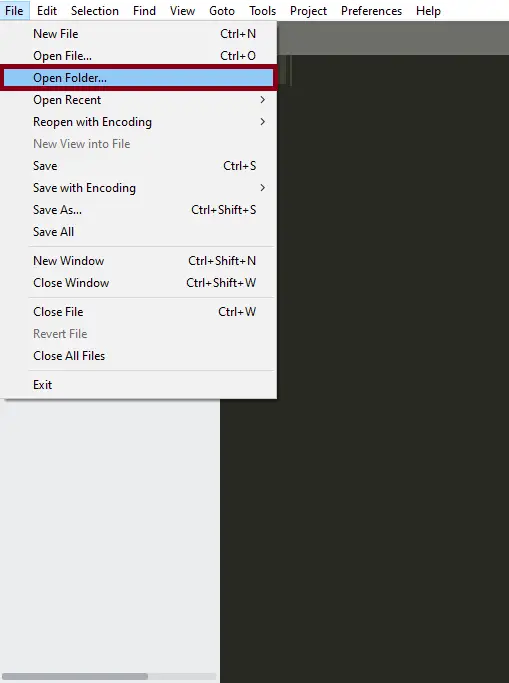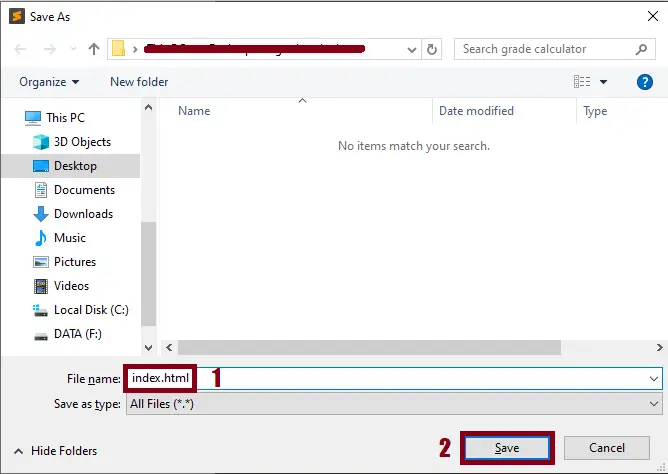# Hangman Game Javascript From Scratch With Source Code

## Hangman Game Javascript From Scratch With Source Code

The Hangman Game Javascript From Scratch was developed using JavaScriptCSS and HTML, In this Simple Javascript Game Project With Source Code you will learn on how to make hangman in javascript. Just follow the instructions below and download the source code.

A Hangman In Javascript is a word-guessing game. One player picks a secret word, and the other player tries to guess it. The number of incorrect guesses before the game ends is up to the players, but completing a character in a noose provides a minimum of six wrong answers until the game ends. The first player to guess the correct answer thinks of the word for the next game.

I have here a suggested list of Best JavaScript Projects with Source Code Free to download and I’m sure this can help you to improve your skills in JavaScript programming and web development as a whole.

To start executing a Hangman Game Javascript From Scratch With Source Code,  makes sure that you have any platform in creating a JavaScriptCSS,  bootstrap, and HTML installed in your computer, in my case I will use Sublime Text.

## Hangman Game Javascript From Scratch With Source Code : Steps on how to create the project

Time needed: 5 minutes

These are the steps on how to create Hangman Game Javascript From Scratch With Source Code

• Step 1: Create a folder.

First, create a folder and named it.• Step 2: Open the folder in “Sublime Text”.

Second ,after creating a folder, open it in “sublime text“.• Step 3: Create a html file.

Third, create a “html” file and save it as “index.html## The code given below is for the html module

```<FORM NAME="f">
<center>
<h1>Hangman Game In Javascript (IT SOURCECODE)</h1>
<TABLE BGCOLOR=red BORDER=1>
<TR>
<TD COLSPAN=4 ALIGN=RIGHT>
Score : <INPUT TYPE=TEXT NAME="score" VALUE="0" onfocus="score.blur();" SIZE=2>
<BR>
Fails (6): <INPUT TYPE=TEXT NAME="lives" VALUE="0" onfocus="lives.blur();" SIZE=2>
</TD>
<TD COLSPAN=7 ALIGN=CENTER>
<INPUT TYPE=TEXT NAME="word" VALUE="    --- Hangman ---" onfocus="word.blur();" SIZE=25>
<BR>
<INPUT TYPE=TEXT NAME="tried" VALUE="Click GO to get a word." onfocus="tried.blur();" SIZE=25>
</TD>
<TD COLSPAN=2 ALIGN=CENTER>
<INPUT TYPE=BUTTON onclick="new_word(this.form);" VALUE=" GO ">
</TD>
</TR>
<TR>
<TD><INPUT TYPE=BUTTON VALUE=" A "   onclick="seek('A');"></TD>
<TD><INPUT TYPE=BUTTON VALUE=" B "   onclick="seek('B');"></TD>
<TD><INPUT TYPE=BUTTON VALUE=" C "   onclick="seek('C');"></TD>
<TD><INPUT TYPE=BUTTON VALUE=" D "   onclick="seek('D');"></TD>
<TD><INPUT TYPE=BUTTON VALUE=" E "   onclick="seek('E');"></TD>
<TD><INPUT TYPE=BUTTON VALUE=" F "   onclick="seek('F');"></TD>
<TD><INPUT TYPE=BUTTON VALUE=" G "   onclick="seek('G');"></TD>
<TD><INPUT TYPE=BUTTON VALUE=" H "   onclick="seek('H');"></TD>
<TD><INPUT TYPE=BUTTON VALUE=" I   " onclick="seek('I');"></TD>
<TD><INPUT TYPE=BUTTON VALUE=" J  "  onclick="seek('J');"></TD>
<TD><INPUT TYPE=BUTTON VALUE=" K "   onclick="seek('K');"></TD>
<TD><INPUT TYPE=BUTTON VALUE=" L  "  onclick="seek('L');"></TD>
<TD><INPUT TYPE=BUTTON VALUE=" M "   onclick="seek('M');"></TD>
</TR>
<TR>
<TD><INPUT TYPE=BUTTON VALUE=" N "   onclick="seek('N');"></TD>
<TD><INPUT TYPE=BUTTON VALUE=" O "   onclick="seek('O');"></TD>
<TD><INPUT TYPE=BUTTON VALUE=" P "   onclick="seek('P');"></TD>
<TD><INPUT TYPE=BUTTON VALUE=" Q "   onclick="seek('Q');"></TD>
<TD><INPUT TYPE=BUTTON VALUE=" R "   onclick="seek('R');"></TD>
<TD><INPUT TYPE=BUTTON VALUE=" S "   onclick="seek('S');"></TD>
<TD><INPUT TYPE=BUTTON VALUE=" T "   onclick="seek('T');"></TD>
<TD><INPUT TYPE=BUTTON VALUE=" U "   onclick="seek('U');"></TD>
<TD><INPUT TYPE=BUTTON VALUE=" V "   onclick="seek('V');"></TD>
<TD><INPUT TYPE=BUTTON VALUE=" W "   onclick="seek('W');"></TD>
<TD><INPUT TYPE=BUTTON VALUE=" X  "  onclick="seek('X');"></TD>
<TD><INPUT TYPE=BUTTON VALUE=" Y "   onclick="seek('Y');"></TD>
<TD><INPUT TYPE=BUTTON VALUE=" Z  "  onclick="seek('Z');"></TD>
</TR>
</TABLE>
</center>
</FORM>```

In this module which is the html module of the simple project.

## The code given below is for the javascript module

```<SCRIPT LANGUAGE="javascript">

/*
Script by Mike Mcgrath- http://website.lineone.net/~mike_mcgrath
Featured on JavaScript Kit (http://javascriptkit.com)
For this and over 400+ free scripts, visit http://javascriptkit.com
*/

var alpha=new Array();
var alpha_index=0;

var bravo=new Array();
var bravo_index=0;

var running=0;
var failnum=0;

function pick()
{
var choice="";
var blank=0;

for (i=0; i<words[index].length; i++)
{
t=0;
for(j=0; j<=alpha_index; j++)
if(words[index].charAt(i)==alpha[j] || words[index].charAt(i)==alpha[j].toLowerCase()) t=1;

if (t) choice+=words[index].charAt(i)+" ";
else
{
choice+="_ ";
blank=1;
}
}

document.f.word.value=choice;

if (!blank)
{
document.f.tried.value="   === You Win! ===";
document.f.score.value++;
running=0;
}
}

function new_word(form)
{
if(!running)
{
running=1;
failnum=0;
form.lives.value=failnum;
form.tried.value="";
form.word.value="";
index=Math.round(Math.random()*10000) % 100;
alpha=words[index].charAt(0);
alpha=words[index].charAt(words[index].length-1);
alpha_index=1;
bravo=words[index].charAt(0);
bravo=words[index].charAt(words[index].length-1);
bravo_index=1;
pick();
}
}

function seek(letter)
{
if (!running) advise(".....Click GO to start !");
else
{
t=0;
for (i=0; i<=bravo_index; i++)
{
if (bravo[i]==letter || bravo[i]==letter.toLowerCase()) t=1;
}

if (!t)
{
document.f.tried.value+=letter+" "
bravo_index++;
bravo[bravo_index]=letter;

for(i=0;i<words[index].length;i++)
if(words[index].charAt(i)==letter || words[index].charAt(i)==letter.toLowerCase()) t=1;

if(t)
{
alpha_index++;
alpha[alpha_index]=letter;
}
else failnum++;

document.f.lives.value=failnum;
if (failnum==6)
{
document.f.tried.value="You lose - Try again!";
document.f.word.value=words[index];
document.f.score.value--;
running=0;
}
else pick();
}
}
}

{
{
savetext=document.f.tried.value;
document.f.tried.value=msg;
}
}

</SCRIPT>```

In this module which is the javascript module of the simple project.